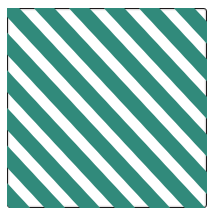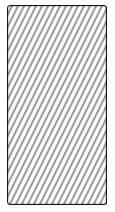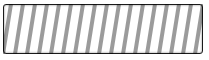# Android Code Snippet[B4X] [B4XCanvas] Fill a panel with diagonally drawn linesUsage:
``FillWithDiagonallyDrawnLines(xpnl_Main,15dip,20dip,xui.Color_ARGB(255,49, 138, 123))``
B4X:
``````Private Sub FillWithDiagonallyDrawnLines(Target As B4XView,Thickness As Float,GapBetween As Float,Color As Int)

Dim Width As Float = Target.Width
Dim Height As Float = Target.Height

Dim xcv As B4XCanvas
xcv.Initialize(Target)

Dim p As Float = -(IIf(Width > Height,Width,Height))-Thickness

Do While p <= Width

xcv.DrawLine(p,0 - (Thickness/2),Width + p,Height + (Thickness/2),Color,Thickness)
p = p + GapBetween + Thickness

Loop

xcv.Invalidate

End Sub``````

Last edited:

#### Alexander Stolte

##### ExpertB4X:
``FillWithDiagonallyDrawnLines2(Pane1,2dip,4dip,xui.Color_ARGB(100,0,0,0))``
B4X:
``````Private Sub FillWithDiagonallyDrawnLines2(Target As B4XView,Thickness As Float,GapBetween As Float,Color As Int)

Dim Width As Float = Target.Width
Dim Height As Float = Target.Height

Dim xcv As B4XCanvas
xcv.Initialize(Target)

Dim p As Float = -(IIf(Width > Height,Width,Height))-Thickness

Do While p <= Width
xcv.DrawLine(p,Height + (Thickness/2),Width + p,0 - (Thickness/2),Color,Thickness)
p = p + GapBetween + Thickness
Loop

xcv.Invalidate

End Sub``````

•LucaMs

#### Alexander Stolte

##### ExpertB4X:
``FillWithDiagonallyDrawnLines3(Pane2,4dip,8dip,xui.Color_ARGB(100,0,0,0))``
B4X:
``````Private Sub FillWithDiagonallyDrawnLines3(Target As B4XView,Thickness As Float,GapBetween As Float,Color As Int)

Dim Width As Float = Target.Width
Dim Height As Float = Target.Height

Dim xcv As B4XCanvas
xcv.Initialize(Target)

Dim p As Float = -(IIf(Width > Height,Width,Height))-Thickness
Do While p <= Width
xcv.DrawLine(p,Target.Height,p+GapBetween,0,Color,Thickness)
p = p + GapBetween + Thickness
Loop

xcv.Invalidate

End Sub``````

•Erel and Johan Hormaza

Replies
14
Views
362
Replies
4
Views
2K
Replies
1
Views
688
Replies
1
Views
1K
Replies
10
Views
12K